# Because of these three knowledge points, I completely abolished the "regular expression"

## Tell a little joke

After finishing work yesterday, it took a long time to get home, because the parking lot downstairs of the company is designed like a maze. It takes a long time to find out that ` I don’t have a car o(╥﹏╥)o.`

` " public account. Maybe you have never met before, but it is very likely that you are too late to meet.`

## `Preface`

`I used to have a sense of fear and aversion to regular expressions. Why? Because I always feel that this stuff is very difficult and boring. Seeing others write thieves, I think when I can be as good as them. Until I saw these three knowledge points. . .`

`Just spend 10 minutes time, you can harvest`

1. ` position matching principle and knowledge in regular expressions `
2. ` string matching principle and knowledge in regular expressions `
3. `The magical use brackets in regular expressions `
4. `14 common regular expression analysis to help understand the knowledge points`

` believe me, after reading this article, you can find solutions and solutions for more than 90% of the regular problems in your work. `

` believe me, after reading this article, you can find solutions and solutions for more than 90% of the regular problems in your work. `

` Believe me, after reading this article, you can find solutions and solutions for more than 90% of the regular problems at work. `

## `Say three silently`

`The regular expression is the matching pattern, either matches the character, or matches the position`

`The regular expression is the matching pattern, either matches the character, or matches the position`

`The regular expression is the matching pattern, either matches the character, or matches the position`

## `1. What can you do to understand the location?`

`Topic 1: Thousandth Division of Numbers`

`Convert 123456789 to 123,456,789`

`Topic 2: Cell phone number 3-4-4 segmentation`

`Convert the mobile phone number 18379836654 to 183-7983-6654`

`Topic 3: Verify the legitimacy of the password`

`The password length is 6-12 digits and consists of numbers, lowercase characters and uppercase letters, but must include at least 2 types of characters`

`These questions often appear in interviews, and they are indispensable in daily business. position, not only can get the interview, you will also write about the business `

### `What is location?`

`Regular expressions are matching patterns, either matching characters or matching positions. So what is position ?`

`FIG follows arrow, the position is understood to a position between adjacent characters .`

`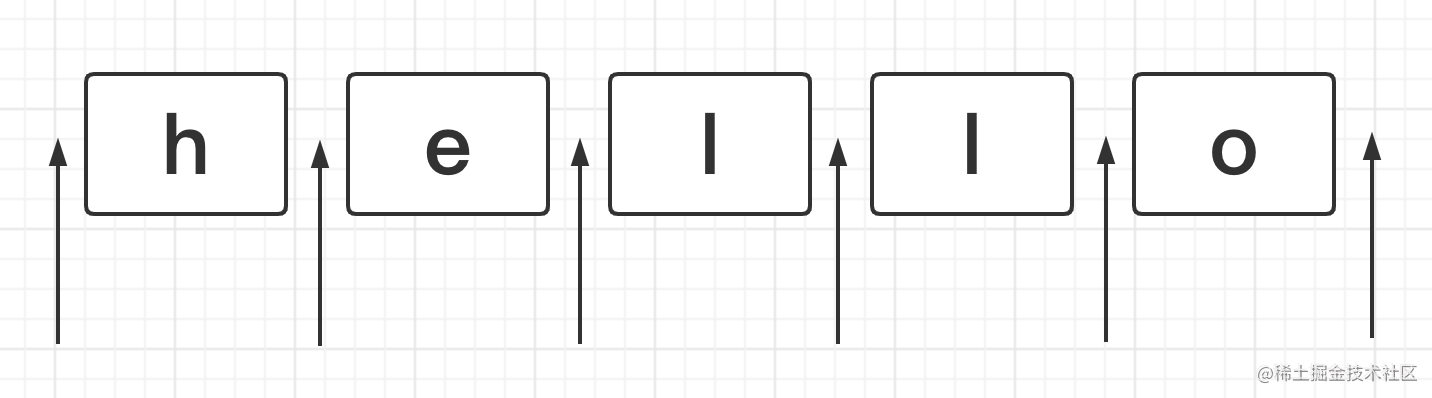`

`We can empty string , the beginning and end of the character, the gap can be connected with an empty string.`

``````'hello' === '' + 'h' + '' + 'e' + '' + 'l' + '' +  'l' + '' + 'o' + '' // true
``````

`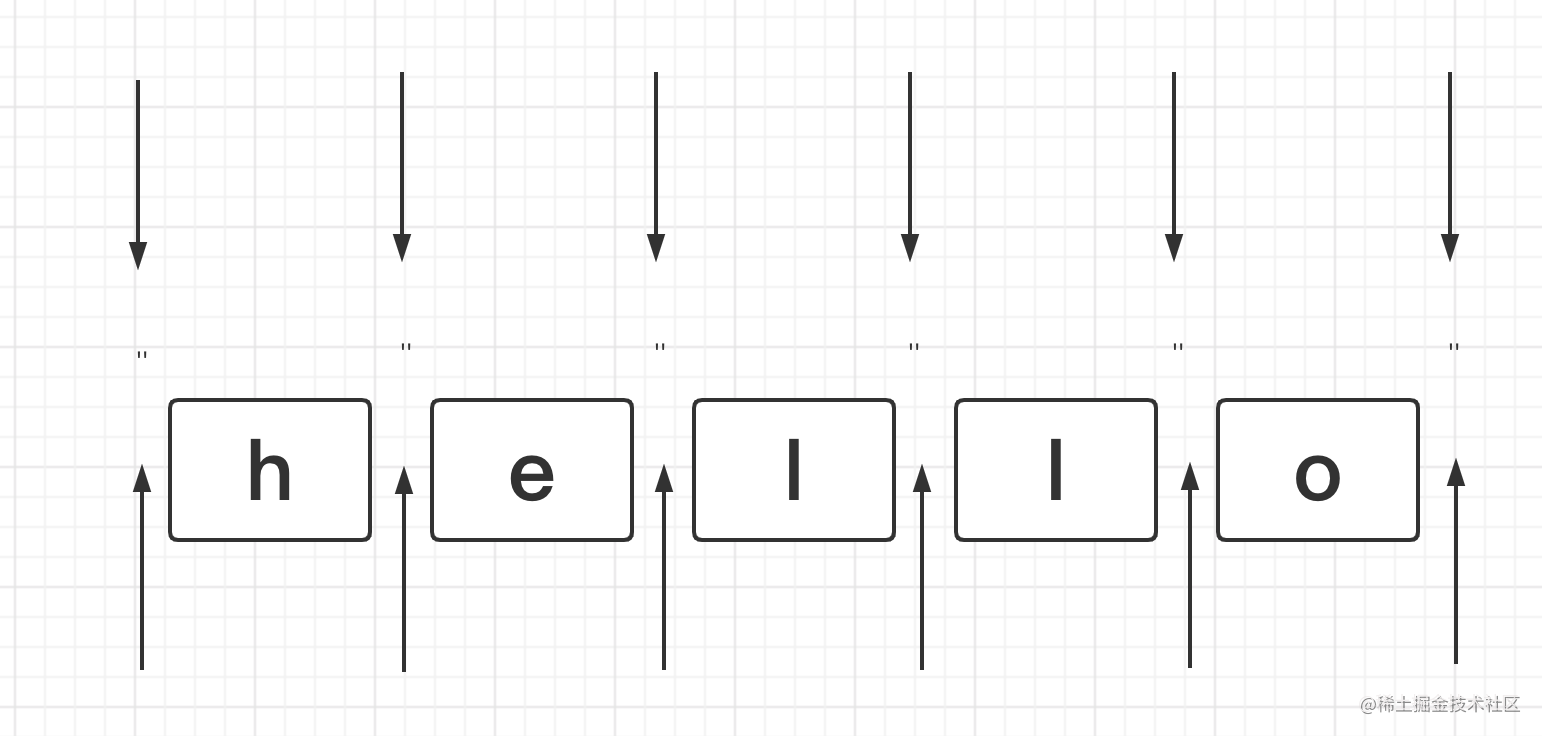`

### `What are the locations?`

`The symbols commonly used to indicate position in regularization mainly include:`

`^、\$、\b、\B、?=p、(?!p)、(?<=p)、(?<!p)`

`Next, let's understand them all one by one.`

#### `^`

`Caret, matches the beginning of the line`

`For example, if you want to put a smiley face (😄) at the beginning of hello, this will definitely not trouble you`

``````
let string = 'hello'

console.log(string.replace(/^/, '😄')) // 😄hello

``````

#### `\$`

`Dollar sign, matching the end of the line`

`Put a smiley face (😄) at the end of hello with ideals?`

``````
let string = 'hello'

console.log(string.replace(/\$/, '😄')) // hello😄

``````

`I believe everyone must be familiar with these two symbols indicating the first and last positions.`

#### `\b`

`There are three rules for the boundaries of words.`

`① The position between \w and \W`

`② The position between ^ and \w`

`③ The position between \w and \$`

`For example, the seed of a certain episode hidden in the learning tutorial xxx_love_study_1.mp4 , how do you want to turn it into ❤️xxx_love_study_1❤️.❤️mp4❤️`

``

`In fact, it only needs to execute one line of code`

``````
'xxx_love_study_1.mp4'.replace(/\b/g, '❤️') // ❤️xxx_love_study_1❤️.❤️mp4❤️
``````

`Drawing understanding is`

`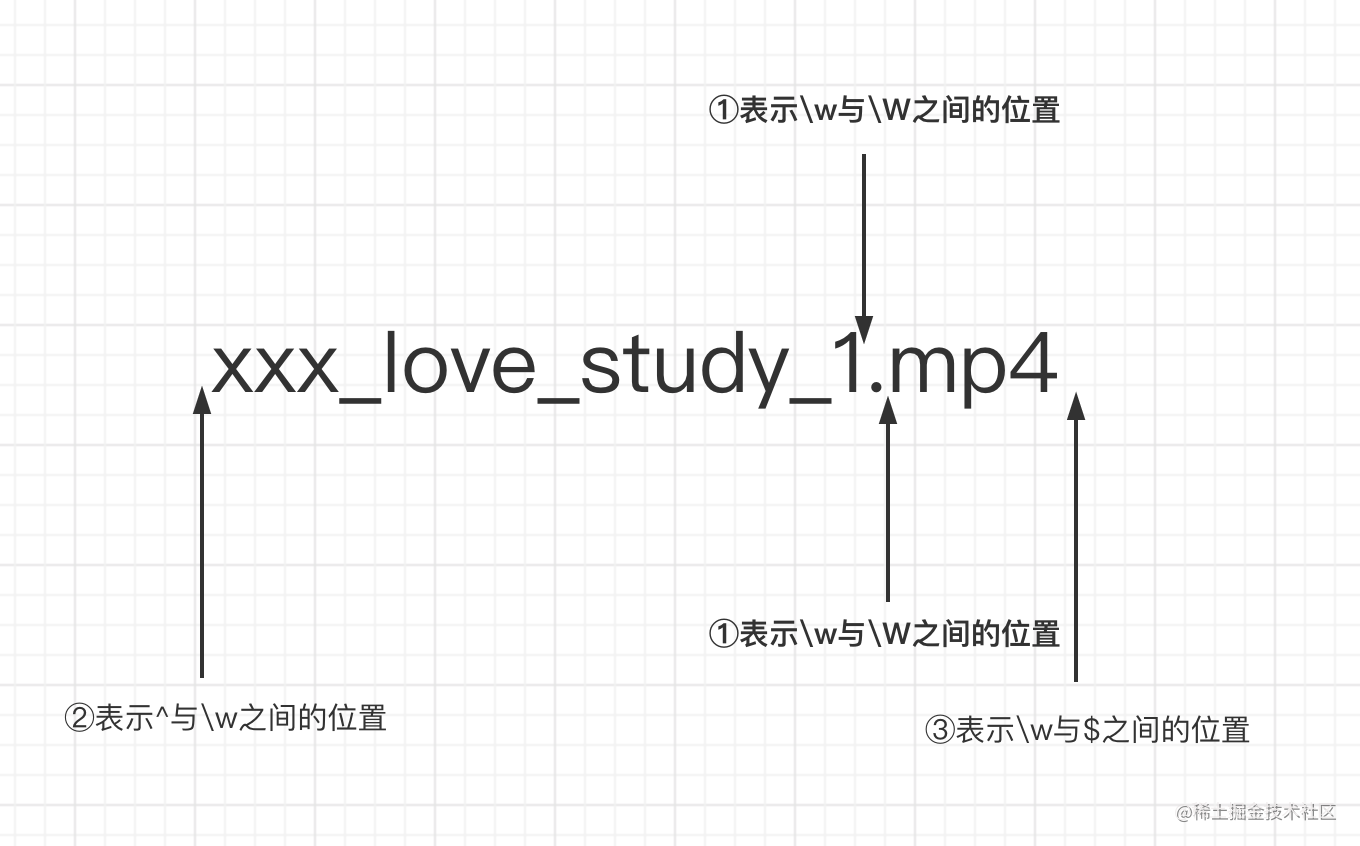`

#### `\B`

`The boundary of a non-word, which is the opposite of \b, has the following rules:`

`① The position between \w and \w`

`② The position between \W and \W`

`③The position between ^ and \W`

`④ The position between \W and \$`

`Also use learn the seeds in the tutorial folder, and make a little modification. After executing this line of code, what will be output?`

``````'[[xxx_love_study_1.mp4]]'.replace(/\B/g, '❤️')
``````

`....`

`That's right, it's full of love! ! ! , Almost can't see the name clearly.`

``````
❤️[❤️[x❤️x❤️x❤️_❤️l❤️o❤️v❤️e❤️_❤️s❤️t❤️u❤️d❤️y❤️_❤️1.m❤️p❤️4]❤️]❤️
``````

`The drawing is explained as follows`

`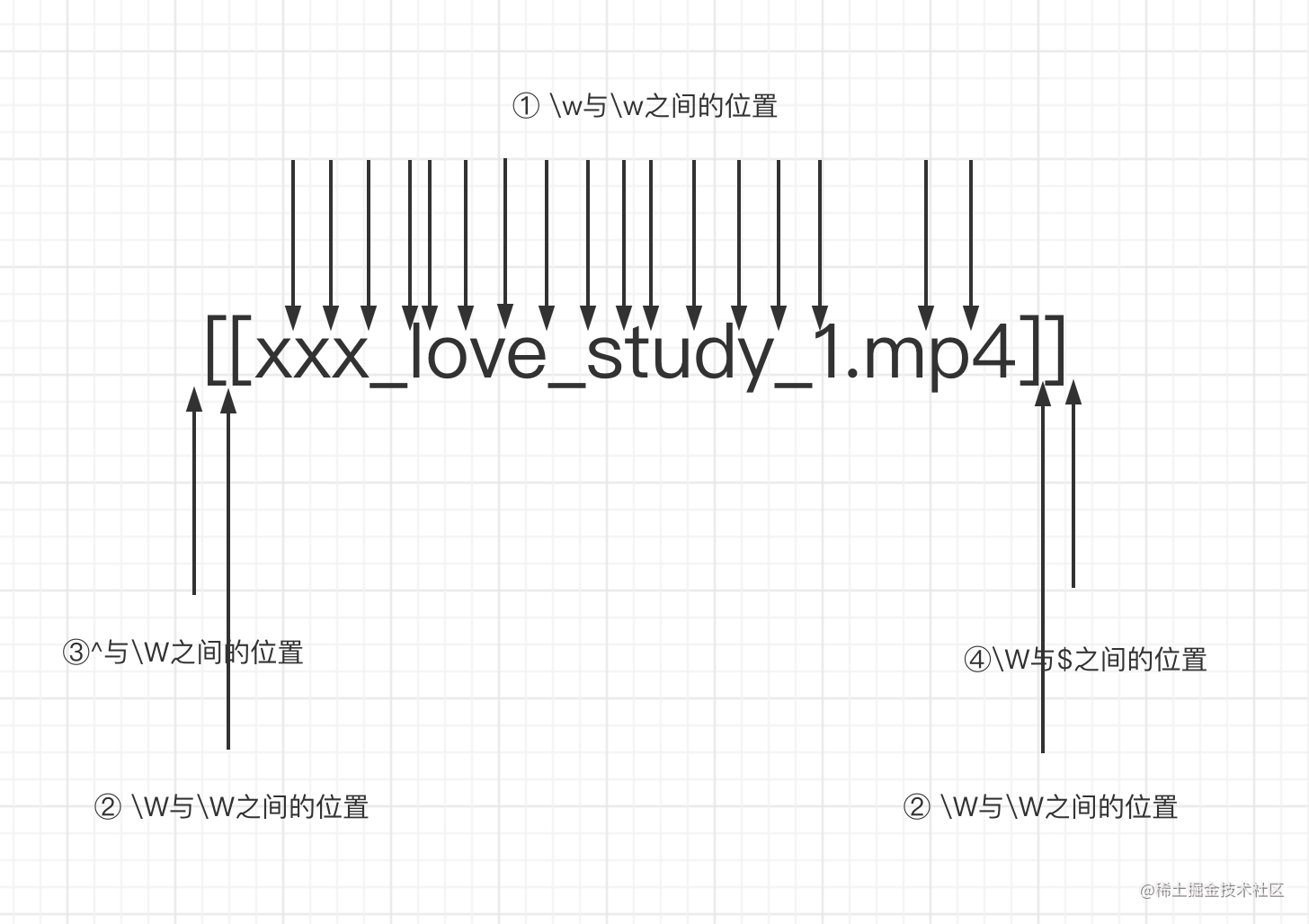`

#### `(?=p)`

`It matches the position in front of the p sub-pattern. In other words, there is a position that needs to satisfy the p sub-pattern immediately after it. There is also a scientific name called positive lookahead assertion.`

`Or this example xxx_love_study_1.mp4 xxx (xxx can refer to any TA you like), how to write it?`

`Is that right? No, this will cause your xxx to disappear, so what else should I do?`

``````
'xxx_love_study_1.mp4'.replace('xxx', '❤️') // ❤️_love_study_1.mp4

``````

`This can be very convenient by using (?=p) (can you think about the difference with the above?)`

``````
'xxx_love_study_1.mp4'.replace(/(?=xxx)/g, '❤️') // ❤️xxx_love_study_1.mp4

``````

`Drawing comprehension`

`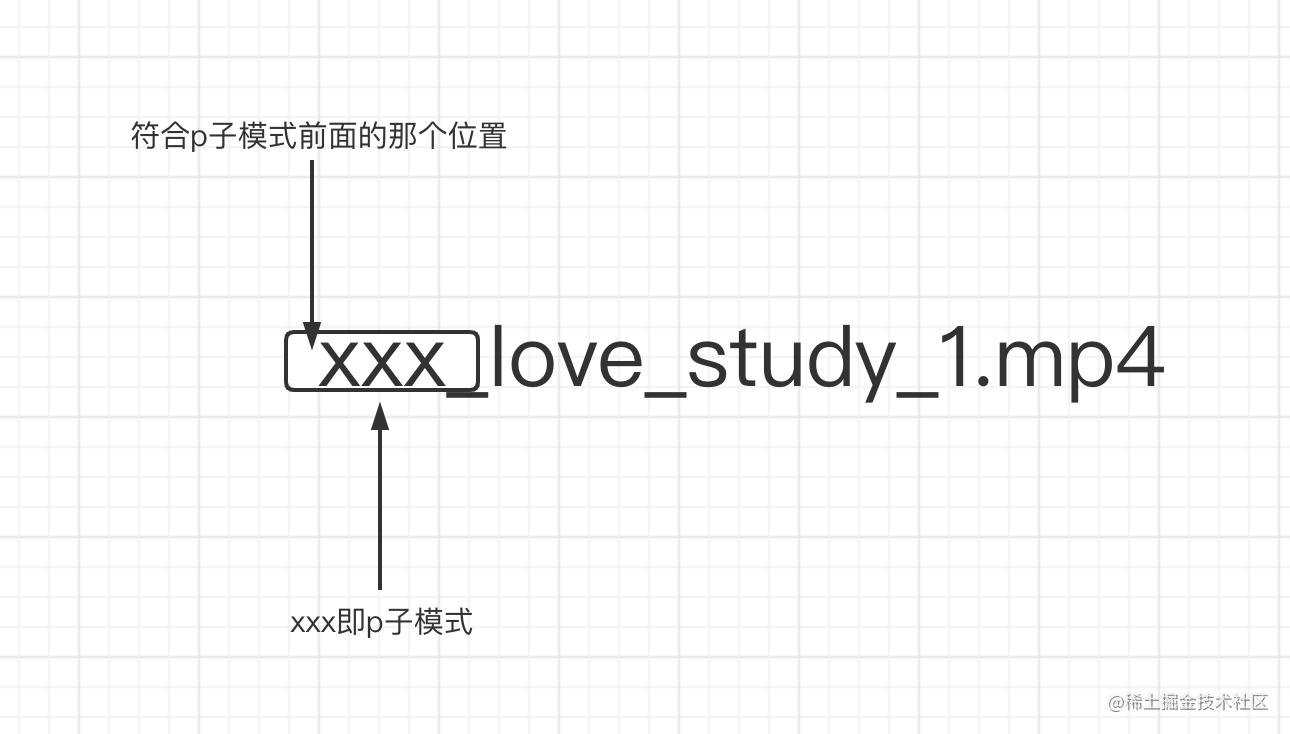`

#### `(?!p)`

`The reverse meaning of (?=p) can be understood as the position other than the position matched by (?=p) belongs to (?!p), and it also has a scientific name called negative lookahead assertion.`
``````
'xxx_love_study_1.mp4'.replace(/(?!xxx)/g, '❤️')

// (?=xxx)的输出
❤️xxx_love_study_1.mp4
// (?!xxx)的输出
x❤️x❤️x❤️_❤️l❤️o❤️v❤️e❤️_❤️s❤️t❤️u❤️d❤️y❤️_❤️1❤️.❤️m❤️p❤️4❤️
``````

`Make a careful comparison. Except for (?=xxx) matched to the front position, the other positions are matched by (?!xxx).`

#### `(?<=p)`

`Meet the position behind the p sub-pattern (note that (?=p) means the front). In other words, there is a position whose front part needs to satisfy the p sub-pattern.`

`This is still the example: we have to put a ❤️ after xxx (xxx can refer to any TA you like), how to write it?`

``````'xxx_love_study_1.mp4'.replace(/(?<=xxx)/g, '❤️') //xxx❤️_love_study_1.mp4

``````

`Drawing explanation`

`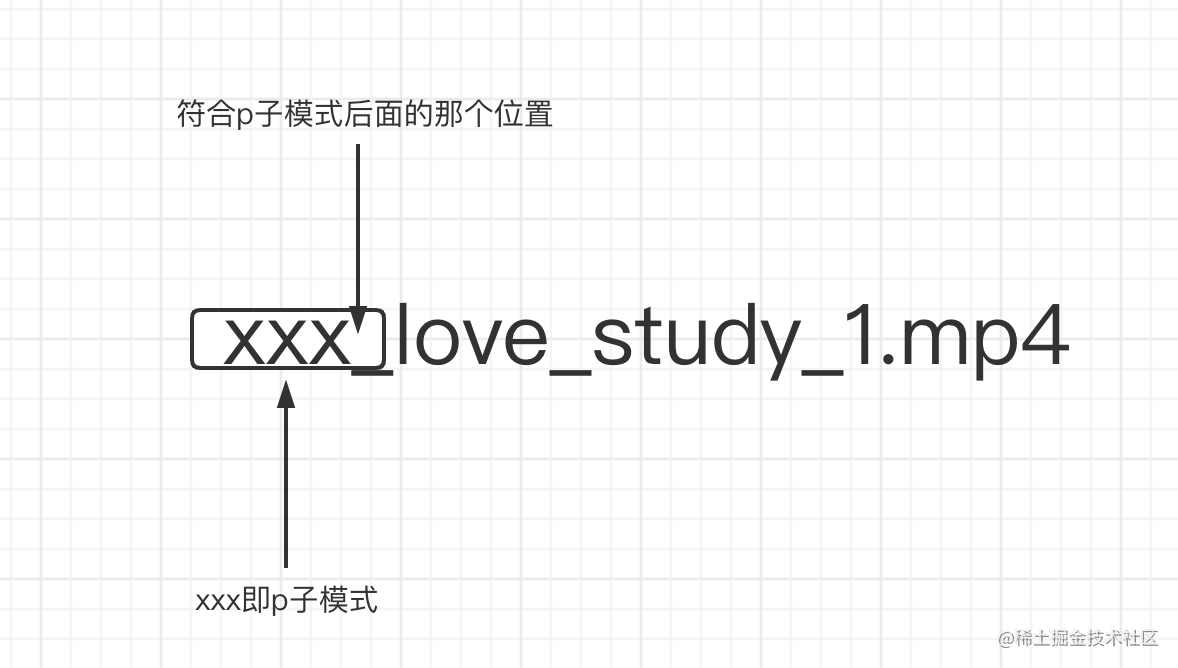`

#### `(?<!p)`

`(?<=p) The converse meaning can be understood as the position other than the matched position of (?<=p) belongs to (?<!p),`
``````
'xxx_love_study_1.mp4'.replace(/(?<!xxx)/g, '❤️')

// (?<=xxx)的输出
xxx❤️_love_study_1.mp4
// (?<!xxx)的输出
❤️x❤️x❤️x_❤️l❤️o❤️v❤️e❤️_❤️s❤️t❤️u❤️d❤️y❤️_❤️1❤️.❤️m❤️p❤️4❤️
``````

`Make a careful comparison. Except for (?<=xxx) matched to the latter position, all other positions are matched by (?<!xxx).`

### `Chestnut detailed`

`After learning the position-related knowledge, let’s try the first few questions`

#### `Topic 1: Thousandth Division of Numbers`

`Convert 123456789 to 123,456,789`

`The rule of observing the question is from back to front, add a comma in front of every three numbers, (note that there is no need to add a comma at the beginning). Is it very consistent?What is the law of (?=p)? p can represent every three digits, and the position of the comma to be added is exactly the position matched by (?=p).`

` first step, try to get the first comma out `

``````

let price = '123456789'
let priceReg = /(?=\d{3}\$)/

console.log(price.replace(priceReg, ',')) // 123456,789

``````

` second step, get all the commas out `

`To get all the commas out, the main problem to be solved is how to represent a group of three numbers , which is a multiple of 3. We know that regular brackets can turn a p pattern into a small whole, so using the nature of brackets, we can write`

``````

let price = '123456789'
let priceReg = /(?=(\d{3})+\$)/g

console.log(price.replace(priceReg, ',')) // ,123,456,789
``````

` third step, remove the first comma, `

`The above has basically fulfilled the requirements, but it is not enough. The first position will appear, so how to remove the first comma? Think about whether there is a piece of knowledge that just satisfies this scene? That's right (?! p), it's him. The combination of the two is to add a comma before every three digits from the back to the front, but this position cannot be the first ^.`

``````
let price = '123456789'
let priceReg = /(?!^)(?=(\d{3})+\$)/g

console.log(price.replace(priceReg, ',')) // 123,456,789``````

#### `Topic 2: Cell phone number 3-4-4 segmentation`

`Convert the mobile phone number 18379836654 to 183-7983-6654`

`With the above number of thousandths division method, I believe it will be much easier to do this question, that is, to find such a position from the back to the front:`

`The position before every four digits, and replace this position with-`

``````
let mobile = '18379836654'
let mobileReg = /(?=(\d{4})+\$)/g

console.log(mobile.replace(mobileReg, '-')) // 183-7983-6654
``````

#### `Topic 3: Mobile phone number 3-4-4 segmentation extension`

`Convert numbers within 11 digits of mobile phone number into 3-4-4 format`

`Recall this scenario. There is a form that needs to collect the user's mobile phone number. The user enters the number one by one. We need to convert it into a 3-3-4 format when the user enters the 11-digit mobile phone number. which is`

``````123 => 123
1234 => 123-4
12345 => 123-45
123456 => 123-456
1234567 => 123-4567
12345678 => 123-4567-8
123456789 => 123-4567-89
12345678911 => 123-4567-8911
``````

`It is not appropriate to use (?=p), for example, 1234 will become -1234.Think about the previous knowledge points that are suitable for handling this kind of scene? Yes(?<=p)`

` first step is to get the first one- `

``````const formatMobile = (mobile) => {
return String(mobile).replace(/(?<=\d{3})\d+/, '-')
}

console.log(formatMobile(123)) // 123
console.log(formatMobile(1234)) // 123-4
``````

` the second one out `

`After getting the first-out, the length of the character is one more bit. Originally 1234567 (insert -)8 in this position, it should be moved one bit backward`

``````const formatMobile = (mobile) => {
return String(mobile).slice(0,11)
.replace(/(?<=\d{3})\d+/, (\$0) => '-' + \$0)
.replace(/(?<=[\d-]{8})\d{1,4}/, (\$0) => '-' + \$0)
}

console.log(formatMobile(123)) // 123
console.log(formatMobile(1234)) // 123-4
console.log(formatMobile(12345)) // 123-45
console.log(formatMobile(123456)) // 123-456
console.log(formatMobile(1234567)) // 123-4567
console.log(formatMobile(12345678)) // 123-4567-8
console.log(formatMobile(123456789)) // 123-4567-89
console.log(formatMobile(12345678911)) // 123-4567-8911
``````

#### `Topic 4: Verify the legitimacy of the password`

`The password length is 6-12 digits and consists of numbers, lowercase characters and uppercase letters, but must include at least 2 types of characters`

`The topic consists of three conditions`

`① The password length is 6-12 digits`

`② Composed of numbers, lowercase characters and uppercase letters`

`③ Must include at least 2 kinds of characters`

` first step is to write the conditions ① and ② and the regular `

``````let reg = /^[a-zA-Z\d]{6,12}\$/
``````

` second step must contain certain characters (digits, lowercase letters, uppercase letters) `

``````let reg = /(?=.*\d)/
// 这个正则的意思是，匹配的是一个位置，这个位置需要满足`任意数量的符号，紧跟着是个数字`，注意它最终得到的是个位置，而不是数字或者是数字前面有任意的东西

console.log(reg.test('hello')) // false
console.log(reg.test('hello1')) // true
console.log(reg.test('hel2lo')) // true

// 其他类型同理
``````

` third step, write the complete regular `

`Must contain two characters, there are the following four permutations and combinations`

`① Combination of numbers and lowercase letters`

`② Combination of numbers and capital letters`

`③ Combination of lowercase and uppercase letters`

`④ Combine numbers, lowercase letters, and uppercase letters together (but in fact, the first three have covered the fourth one)`

``````// 表示条件①和②
// let reg = /((?=.*\d)((?=.*[a-z])|(?=.*[A-Z])))/
// 表示条件条件③
// let reg = /(?=.*[a-z])(?=.*[A-Z])/
// 表示条件①②③
// let reg = /((?=.*\d)((?=.*[a-z])|(?=.*[A-Z])))|(?=.*[a-z])(?=.*[A-Z])/
// 表示题目所有条件
let reg = /((?=.*\d)((?=.*[a-z])|(?=.*[A-Z])))|(?=.*[a-z])(?=.*[A-Z])^[a-zA-Z\d]{6,12}\$/

console.log(reg.test('123456')) // false
console.log(reg.test('aaaaaa')) // false
console.log(reg.test('AAAAAAA')) // false
console.log(reg.test('1a1a1a')) // true
console.log(reg.test('1A1A1A')) // true
console.log(reg.test('aAaAaA')) // true
console.log(reg.test('1aA1aA1aA')) // true
``````

## `2. String matching turns out to be so simple`

### `Two kinds of fuzzy matching`

`If there is only an exact match, then it is completely meaningless`

#### `Horizontal`

`The length of a string that can be matched by a regular pattern is not fixed, and it can be in a variety of situations. Through the quantifiers +, *,?, {m,n}, horizontal matching can be achieved`
``````let reg = /ab{2,5}c/
let str = 'abc abbc abbbc abbbbc abbbbbc abbbbbbc'

str.match(reg) // [ 'abbc', 'abbbc', 'abbbbc', 'abbbbbc' ]``````

#### `Vertical`

`A regular matching string, specific to a certain character, may not be a certain string, there can be many possibilities, the realization method is a character group (in fact, multi-choice branch|can also be realized)`
``````let reg = /ac/
let str = 'a0b a1b a2b a3b a4b'

str.match(reg) // [ 'a1b', 'a2b', 'a3b' ]``````

### `Character set`

`Don’t be fooled by the name. Although it is called a character group, it actually represents the possibility of a character.`

#### `Range notation`

``[123456abcdefABCDEF] => [1-6a-fA-F]``

#### `Exclude character groups`

`A character can be anything, but it cannot be xxx, use the ^ symbol`

`Question: How to represent anything except a certain word?`

``[^abc]``

#### `Common abbreviations`

``````\d // 数字
\D // 非数字
\w // [0-9a-zA-Z_]
\W // [^0-9a-zA-Z_]
\s // [\t\v\n\r\f]
\S // [^\t\v\n\r\f]
.``````

### `quantifier`

#### `Quantifiers & Abbreviations`

``````1. {m,} // 至少出现m次
2. {m} // 出现m次
3. ? // 出现0次或者1次，等价于{0,1}
4. + // 至少出现1次,等价于{1,}
5. * // 出现人一次,等价于{0,}  ``````

#### `Greedy matching VS lazy matching`

`The regular itself is greedy and will match as many characters as possible that match the pattern`
``````let regex = /\d{2,5}/g
let string = '123 1234 12345 123456'
// 贪婪匹配
// string.match(regex) // [ 123, 1234, 12345, 12345 ]

// 惰性匹配
let regex2 = /\d{2,5}?/g
// string.match(regex) // [ 12, 12, 34, 12, 34, 12, 34, 56  ]``````

`Add one after the quantifier? , Which becomes a lazy match`

``````贪婪量词        惰性量词
{m,n}            {m,n}?
{m,}             {m,}?
?                       ??
+                       +?
*                   *?  ``````

### `Multiple choice branch`

`One mode can achieve horizontal and vertical fuzzy matching, and the multi-choice branch can support multiple sub-modes to choose one, the form is (p1|p2|p3)`

``````let regex = /good|nice/
let string = 'good idea, nice try.'

// string.match(regex) // [ 'good', 'nice' ]

// 注意，用/good|goodbye/去匹配'goodbye' 匹配到的是good
// 因为分支结构是惰性的，前面的匹配上了，后面的就不再尝试了``````

### `case analysis`

#### `1. Match id`

``````// 1
let regex = /id=".*?"/ // 想想为什么要加? 不加的话 连后面的class都会匹配到
let string = '<div id="container" class="main"></div>';
console.log(string.match(regex));
// 2
let regex = /id="[^"]*"/
let string = '<div id="container" class="main"></div>';
console.log(string.match(regex)); ``````

#### `2. Match the hexadecimal color value`

``````// 要求匹配如下颜色
/*
#Fc01DF
#FFF
#ffE
*/

let regex = /#([a-fA-F\d]{6}|[a-fA-F\d]{3})/g
let string = "#ffbbad #Fc01DF #FFF #ffE";

console.log(string.match(regex))

#### `3. Match the 24-hour system time`

``````/*
要求匹配
23:59
02:07
*/
// 解析：
// 第一位：可以是0、1、2
// 第二位：当第一位位0或者1的时候，可以是0到9、第一位是2的时候，只可以是0到3
// 第三位：固定是冒号：
// 第四位：可以是0到5
// 第五位：0到9

let regex = /^(\d|2[0-3]):[0-5]\d\$/

console.log(regex.test('23:59')) // true
console.log(regex.test('02:07'))// true

// 衍生题，可以是非0
let regex = /^(0?\d|1\d|2[0-3]):(0?|[1-5])\d/

console.log( regex.test("23:59") ) // true
console.log( regex.test("02:07") ) // true
console.log( regex.test("7:09") ) // true``````

#### `4. Match date`

``````/*
要求匹配
yyyy-mm-dd格式的日期
注意月份、和日的匹配
*/

let regex = /\d{4}-(0\d|1[0-2])-(0[1-9]|\d|3)/

console.log( regex.test("2017-06-10") ) // true
console.log( regex.test("2017-11-10") ) // true``````

## `3. The magical effect of parentheses`

`The function of the parentheses is to provide grouping (the regularity in the parentheses is a whole, that is, to provide sub-expression), so that we can refer to it`

#### `Grouping`

`How to make the quantifier work on a whole?`

``````let reg = /(ab)+/g
let string = 'ababa abbb ababab'

console.log(string.match(reg)) // ["abab", "ab", "ababab"]``````

#### `Branch structure`

`The branch structure is a bit like the concept of or in programming ||`

``````/*

I love JavaScript
I love Regular Expression
*/

let reg = /I love (JavaScript|Regular Expression)/

console.log(reg.test('I love JavaScript')) // true
console.log(reg.test('I love Regular Expression')) // true``````

### `Group reference`

`Create sub-expression through parentheses, data extraction and powerful replacement operations can be performed, and grouping content can also be referenced through js`

#### `Extract data`

``````/*

2021-08-14
*/

let reg = /(\d{4})-(\d{2})-(\d{2})/

console.log('2021-08-14'.match(reg))
//  ["2021-08-14", "2021", "08", "14", index: 0, input: "2021-08-14", groups: undefined]

// 第二种解法,通过全局的\$1...\$9读取 引用的括号数据
let reg = /(\d{4})-(\d{2})-(\d{2})/
let string = '2021-08-14'

reg.test(string)

console.log(RegExp.\$1) // 2021
console.log(RegExp.\$2) // 08
console.log(RegExp.\$3) // 14``````

#### `Data replacement`

``````/*

2021-08-14
*/
// 第一种解法
let reg = /(\d{4})-(\d{2})-(\d{2})/
let string = '2021-08-14'
// 第一种写法
let result1 = string.replace(reg, '\$2/\$3/\$1')
console.log(result1) // 08/14/2021
// 第二种写法
let result2 = string.replace(reg, () => {
return RegExp.\$2 + '/' + RegExp.\$3 + '/' + RegExp.\$1
})
console.log(result2) // 08/14/2021
// 第三种写法
let result3 = string.replace(reg, (\$0, \$1, \$2, \$3) => {
return \$2 + '/' + \$3 + '/' + \$1
})
console.log(result3) // 08/14/2021``````

### `Back reference (very important)`

`In addition to quoting the content of the group through js, the content of the group can also be referenced through regular`

``````/*
写一个正则支持以下三种格式
2016-06-12
2016/06/12
2016.06-12
*/
let regex = /(\d{4})([-/.])\d{2}\1\d{2}/

var string1 = "2017-06-12";
var string2 = "2017/06/12";
var string3 = "2017.06.12";
var string4 = "2016-06/12";

console.log( regex.test(string1) ); // true
console.log( regex.test(string2) ); // true
console.log( regex.test(string3) ); // true
console.log( regex.test(string4) ); // false``````

#### `Notice`

1. `What happens if you refer to a group that does not exist?`

1. `That is, the match is \1 \2 itself`
2. `What if there are quantifiers behind the group?`

1. `If there is a quantifier after the grouping, the final data captured by the grouping (note that it is a grouping, not the whole) is the last match`
``````'12345'.match(/(\d)+/) // ["12345", "5", index: 0, input: "12345", groups: undefined]

/(\d)+ \1/.test('12345 1') // false
/(\d)+ \1/.test('12345 5') // true``````

### `Non-capturing parentheses`

`The brackets used above will match the data they match for subsequent reference, so it can also be called capture grouping and capture branch.`

`If you want the most primitive function of brackets, but will not quote it, that is, it will neither appear in API references, nor in regular references, you can use`

`Non-capturing parentheses (?:p)`

``````// 非捕获型引用
let reg = /(?:ab)+/g
console.log('ababa abbb ababab'.match(reg)) // ["abab", "ab", "ababab"]
// 注意这里，因为是非捕获型分组，所以使用match方法时，不会出现在数组的1位置了
let reg = /(?:ab)+/
console.log('ababa abbb ababab'.match(reg)) // ["abab", index: 0, input: "ababa abbb ababab", groups: undefined]
let reg = /(ab)+/
console.log('ababa abbb ababab'.match(reg)) // ["abab", "ab", index: 0, input: "ababa abbb ababab", groups: undefined]``````

### `Case study`

#### `1. Trim method simulation`

``````// 1. 提取中间关键字符, 使用的分组引用
const trim1 = (str) => {
return str.replace(/^\s*(.*?)\s*\$/, '\$1')
}
// 2. 去掉开头和结尾的空字符
const trim2 = (str) => {
return str.replace(/^\s*|\s*\$/g, '')
}``````

#### `2. Capitalize the first letter of each word`

`The key is to find the first letter of each word`

``````// my name is epeli

const titleize = (str) => {
return str.toLowerCase().replace(/(?:^|\s)\w/g, (c) => c.toUpperCase())
}

console.log(titleize('my name is epeli')) // My Name Is Epeli

// 拓展，横向转驼峰，例如base-act-tab => BaseActTab
'base-act-tab'.replace(/(?:^|-)(\w)/g, (\$0, \$1) => \$1.toUpperCase()) // BaseActTab``````

#### `3. Humping`

``````// -moz-transform => MozTransform
const camelize = (str) => {
return str.replace(/[-_\s]+(\w)/g, (_, \$1) => \$1.toUpperCase())
}

console.log(camelize('-moz-transform')) // MozTransform``````

#### `4. Underlined`

``````// MozTransform => -moz-transform
const dasherize = (str) => {
return str.replace(/[A-Z]/g, (\$0) => ('-' + \$0).toLowerCase())
}

console.log(dasherize('MozTransform')) // -moz-transform``````

#### `5. HTML Escaping and Contrasting`

``````// html转义规则见https://blog.wpjam.com/m/character-entity/

const escapeHTML = (str) => {
const escapeChars = {
'<': 'lt',
'>': 'gt',
'"': 'quot',
''': '#39',
'&': 'amp'
}

let regexp = new RegExp(`[\${Object.keys(escapeChars).join('')}]`, 'g') // 为了得到字符组[<>"'&]

return str.replace(regexp, (c) => `&\${escapeChars[ c ]};`)
}

console.log( escapeHTML('<div>Blah blah blah</div>')) // &lt;div&gt;Blah blah blah&lt;/div&gt;

// 反转义
const unescapseHTML = (str) => {
const htmlEntities = {
nbsp: ' ',
lt: '<',
gt: '>',
quot: '"',
amp: '&',
apos: '''
}

return str.replace(/&([^;]+);/g, (\$0, \$1) => {
return htmlEntities[ \$1 ] || ''
})
}

console.log(unescapseHTML('&lt;div&gt;Blah blah blah&lt;/div&gt;')) // <div>Blah blah blah</div>``````

#### `6. Match paired tags`

``````/*
匹配
<title>regular expression</title>
<p>laoyao bye bye</p>
不匹配
<title>wrong!</p>
*/
let reg = /<([^>]+)>.*?</\1>/g

console.log(reg.test('<title>regular expression</title>')) // true
console.log(reg.test('<p>laoyao bye bye</div>')) // false``````

### `Meet bye`

`I strongly recommend Yao 's regular expression mini-book . After reading this book, the author slowly began to understand the regularity and no longer resisted it. This article is mainly based on the content of this book. Summarize.`

### `refer to`

1. ` JS regular expression complete tutorial (slightly longer) `
2. ` 30-minute package meeting-regular expression `
3. ` Talk about regular expressions that make people headache `
`前端javascript正则表达式面试vue.js`
`阅读 4.1k发布于 2021-10-22 `
`本作品系原创，采用《署名-非商业性使用-禁止演绎 4.0 国际》许可协议谦龙的学习笔记just go`
`前端胖头鱼`
`3.7k 声望`
`6.1k 粉丝`
`0 条评论`
`推荐阅读`
`面试官：Vue3响应式系统都不会写，还敢说精通？也许你我素未谋面，但很可能相见恨晚，我是前端胖头鱼前言都说今年是最惨工作年，大厂裁员，小厂跟风，简历投了几百封回信的寥寥无几，金三银四怕是成了铜三铁四，冷冷清清，凄凄惨惨。但是今天的主角，小帅同学...前端胖头鱼赞 6阅读 5.6k评论 2ESlint + Stylelint + VSCode自动格式化代码(2023)安装插件 ESLint，然后 File -&gt; Preference-&gt; Settings（如果装了中文插件包应该是 文件 -&gt; 选项 -&gt; 设置），搜索 eslint，点击 Edit in setting.json谭光志赞 34阅读 20.8k评论 9安全地在前后端之间传输数据 - 「3」真的安全吗？在「2」注册和登录示例中，我们通过非对称加密算法实现了浏览器和 Web 服务器之间的安全传输。看起来一切都很美好，但是危险就在哪里，有些人发现了，有些人嗅到了，更多人却浑然不知。就像是给门上了把好锁，还...边城赞 32阅读 7.3k评论 5vue UI框架比较最好基于vue2.0PC端：因为用过的是饿了么UI，所以比较以饿了么UI为基础element UI 饿了么UI支持vue2.x80分优点：组件的API方法、属性等封装的较为完善缺点：样式有些生硬，不够炫酷美观N3 N3支持vue2.x70分优点：...chinawzc赞 22阅读 39.9k评论 17涨姿势了，有意思的气泡 Loading 效果今日，群友提问，如何实现这么一个 Loading 效果：这个确实有点意思，但是这是 CSS 能够完成的？没错，这个效果中的核心气泡效果，其实借助 CSS 中的滤镜，能够比较轻松的实现，就是所需的元素可能多点。参考我们...chokcoco赞 24阅读 2.3k评论 3你可能不需要JS！CSS实现一个计时器CSS现在可不仅仅只是改一个颜色这么简单，还可以做很多交互，比如做一个功能齐全的计时器？样式上并不复杂，主要是几个交互的地方数字时钟的变化开始、暂停操作重置操作如何仅使用 CSS 来实现这样的功能呢？一起...XboxYan赞 25阅读 1.7k评论 1在前端使用 JS 进行分类汇总最近遇到一些同学在问 JS 中进行数据统计的问题。虽然数据统计一般会在数据库中进行，但是后端遇到需要使用程序来进行统计的情况也非常多。.NET 就为了对内存数据和数据库数据进行统一地数据处理，发明了 LINQ (L...边城赞 17阅读 2k`
`4334`